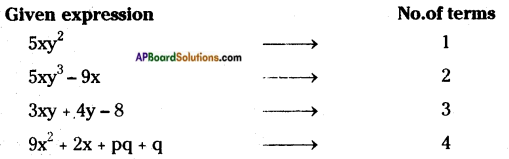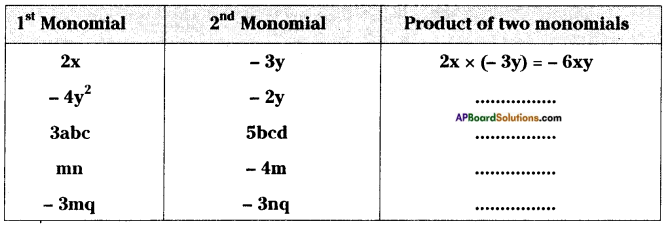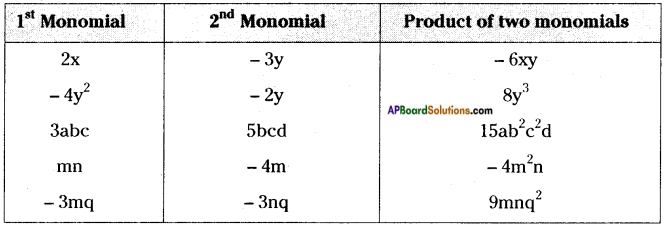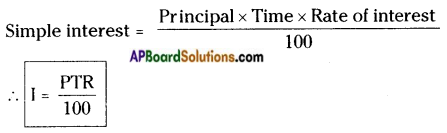# AP Board 8th Class Maths Solutions Chapter 11 Algebraic Expressions InText Questions

## AP State Syllabus 8th Class Maths Solutions 11th Lesson Algebraic Expressions InText Questions

AP State Syllabus AP Board 8th Class Maths Solutions Chapter 11 Algebraic Expressions InText Questions and Answers.

### 8th Class Maths 11th Lesson Algebraic Expressions InText Questions and Answers

Do this

Question 1.
Find the number of terms in following algebraic expressions.
5xy2, 5xy3 – 9x, 3xy + 4y – 8, 9x2 + 2x + pq + q.    [Page No. 248]Question 2.
Take different values for x and find values of 3x + 5.     [Page No. 248]
If x = 1 then 3x + 5 = 3(1) + 5 = 3 + 5 = 8
If x = 2 then 3x + 5 = 3(2) + 5 = 6 + 5 = 11
If x = 3 then 3x + 5 = 3(3) + 5 = 9 + 5 = 14

Question 3.
Find the like terms in the following: ax2y, 2x, 5y2, -9x2, -6x, 7xy, 18y2.    [Pg. No. 249]
Like terms are (2x, – 6x) (5y2, 18y2).

Question 4.
Write 3 like terms for 5pq2.     [Pg. No. 249]
Like terms of 5pq2 are – 3pq2, pq2, $$\frac{\mathrm{pq}^{2}}{2}$$etc.,

Question 5.
If A = 2y2 + 3x – x2, B = 3x2 – y2 and C = 5x2 – 3xy then find          [Pg. No. 250]
(i) A + B (ii) A – B (iii) B + C (iv) B – C (v) A + B + C (vi) A + B – C
A = 2y2 + 3x – x2, B = 3x2 – y2, C = 5x2 – 3xy
i) A + B = (2y2 + 3x – x2) + (3x2 – y2)
= (2y2 – y2) + 3x + (3x2 – x2)
∴ A + B = y2 + 3x + 2x2 = 2x2 + 3x + y2

ii) A – B = (2y2 + 3x – x2) – (3x2 – y2)
= 2y2 + 3x – x2 – 3x2 + y2
∴ A – B = 3y2 + 3x – 4x2

iii) B + C = (3x2 – y2) + (5x2 – 3xy)
= 3x2 + 5x2 – y2 – 3xy
∴ B + C = 8x2 – y2 – 3xy

iv) B – C = (3x2 – y2) – (5x2 – 3xy)
= 3x2 – y2 – 5x2 + 3xy
∴ B – C = – 2x2 – y2 + 3xy

v) A + B + C = A + (B + C)
= (2y2 + 3x – x2) + (8x2 – y2 – 3xy)
= (8x2 – x2) + (2y2 – y2) + 3x – 3xy
∴ A + B + C = 7x2 + y2 + 3x – 3xy

vi) A + B – C = A + (B – C)
= (2y2 + 3x – x2) + (-2x2 – y2 + 3xy)
= (2y2 – y2) + (-x2 – 2x2) + 3x + 3xy :
∴ A + B – C = y2 – 3x2 + 3x + 3xyQuestion 6.
Complete the table:       [Page No. 253]Question 7.
Check whether you always get a monomial when two monomials are multiplied.        [Page No. 253]
Yes, the product of two monomials is always a monomial.
Ex: 2xy × 5y = 10xy is a monomial.

Question 8.
Product of two monomials is a monomial? Check.     [Pg. No. 253]
Yes, the product of two monomials is a monomial.
∵ 2x × y = 2xy

Question 9.
Find the product: (i) 3x(4ax + 8by) (ii) 4a2b(a – 3b) (iii) (p + Sq2) pq (iv) (m3 + n3) 5mn2       [Pg. No. 255]
i) 3x (4ax + 8by) = 3x × 4ax + 3x × 8by
= 12ax2 + 24bxy

ii) 4a2b (a – 3b) = 4a2b × a – 4a2b × 3b
= 4a3b – 12a2b2

iii) (p + 3q2) pq = p × pq + 3q2 × pq
= p2q + 3pq3

iv) (m3 + n3) 5mn2 = m3 × 5mn2 + n3 × 5mn2
= 5m4n2 + 5mn5Question 10.
Find the number of maximum terms in the product of a monomial and a binomial?       [Pg. No. 255]
The no.of terms in the product of a monomial and a binomial are two (2).

Question 11.
Find the product:       [Pg. No. 257]
(i) (a – b) (2a + 4b)
(ii) (3x + 2y) (3y – 4x)
(iii) (2m – l)(2l – m)
(iv) (k + 3m)(3m – k)
i) (a – b) (2a + 4b) = a(2a + 4b) – b(2a + 4b)
= (a × 2a + a × 4b) – (b × 2a + b × 4b)
= 2a2 + 4ab – (2ab + 4b2)
= 2a2 + 4ab – 2ab – 4b2
= 2a2 + 2ab – 4b2

ii) (3x + 2y) (3y – 4x) = 3x(3y – 4x) + 2y(3y – 4x)
= 9xy – 12x2 + 6y2 – 8xy
= xy – 12x2 + 6y2

iii) (2m – l) (2l – m) = 2m(2l – m) – l(2l – m)
= 2m × 2l – 2m × m – l × 2l + l × m
= 4lm – 2m2 – 2l2 + lm
= 5lm – 2m2 – 2l2

iv) (k + 3m) (3m – k) = k(3m – k) + 3m(3m – k)
= k × 3m – k × k + 3m × 3m – 3m × k
= 3km – k2 + 9m2 – 3km
= 9m2 – k2

Question 12.
How many number of terms will be there in the product df two binomials?        [Page No. 257]
No. of terms in the product of two binomials are 4.
Ex: (a + b) (c + d) = ac + ad + be + bdQuestion 13.
Verify the following are identities by taking a, b, c as positive integers.    [Pg. No. 260]
(i) (a – b)2 = a2 – 2ab + b2
(ii) (a + b) (a – b) = a2 – b2
(iii) (a + b + c)2 = a2 + b2 + c2 + 2ab + 2bc + 2ca
i) (a – b)2 = a2 – 2ab + b2
a = 3, b = 1
⇒ (3 – 1)2 = (3)2 – 2 × 3 × 1 + 12
⇒ (2)2 = 9 – 6 + 1
⇒ 4 = 4
∴ (i) is an identity,

ii) (a + b) (a – b) = a2 – b2
a = 2, b = 1
⇒ (2 + 1) (2 – 1) = (2)2 – (1)2
⇒ 3 × 1 = 4 – 1
⇒ 3 = 3
∴ (ii) is an identity.

iii) (a + b + c)2 = a2 + b2 + c2 + 2ab + 2bc + 2ca
a = 1, b = 2, c = 0
⇒ (1 + 2 + 0)2 = 12 + 22 + 02 + 2 × 1 × 2 + 2 × 2 × 0 + 2 × 0 × 1
⇒ (3)2 = 1 + 4 + 0 + 4 + 0 + 0
⇒ 9 = 1 + 4 + 4
⇒ 9 = 9
∴ (iii) is an identity.

Question 14.
Now take x = 2, a = 1 and b = 3, verify the identity (x + a) (x + b) s x + (a + b)x + ab.        [Pg. No. 260]
i) What do you observe? Is LHS = RHS?
ii) Take different values for x, a and b for verification of the above identity.
iii) Is it always LHS = RHS for all values of a and b?
i) (x + a) (x + b) = x2 + (a + b)x + ab
x = 2, a = 1, b = 3 then
⇒ (2 + 1) (2 + 3) = 22 + (1 + 3)2 + 1 × 3
⇒ 3 × 5 = 4 + 4x2 + 3
⇒ 15 = 4 + 8 + 3 ⇒ 15 = 15
∴ LHS = RHS

ii) x = 0, a = 1, b = 2 then
⇒ (0 + 1) (0 + 2) = 02 + (1 + 2) 0 + 1 × 2
⇒ 1 × 2 = 0 + 0 + 2
⇒ 2 = 2
∴ LHS = RHS for different values of x, a, b.

iii) LHS = RHS for all the values of a, b.Question 15.
Consider (x + p) (x + q) = x + (p + q)x + pq.
(i) Put q instead of ‘p’ what do you observe?
(ii) Put p instead of ‘q’ what do you observe?
(iii) What identities you observed in your results?            [Pg. No. 261]
i) (x + p) (x + q) = x2 + (p + q)x + pq …… (1)
Substitute q instead of p in (1).
⇒ (x + q) (x + q) = x2 + (q + q)x + q × q
⇒ (x + q)2 = x2 + 2qx + q2

ii) Substitute ‘p’ instead of q in (1).
⇒ (x + p) (x + p) = x2 + (p + p)x + p × p
⇒ (x + p) = x2 + 2px + p2

iii) ∴ I observe the following identities.
(x + q)2 = x2 + 2qx + q2
(x + p)2 = x2 + 2px + p2

Question 16.
Find: (i) (5m + 7n)2
(ii) (6kl + 7mn)2
(iii) (5a2 + 6b2)2
(iv) 3022
(v) 8072
(vi) 7042
(vii) Verify the identity: (a – b)2 = a2 – 2ab + b2, where a = 3m and b = 5n.         [Pg. No. 261]
i) (5m + 7n)2 is in the form of (a + b)2.
(a + b)2 = a2 + 2ab + b2 [a = 5m, b = 7n]
(5m + 7n)2 = (5m)2 + 2 × 5m × 7n + (7n)2
= (5m × 5m) + 70 mn + 7n × 7n
= 25m2 + 70mn + 49n2

ii) (6kl + 7mn)2
We know that (a + b)2 = a2 + 2ab + b2
∴ (6kl + 7mn)2 = (6kl)2 + 2 × 6kl × 7mn + (7mn)2
= 36 k2l2 + 84 klmn + 49 m2n2

iii) (5a2 + 6b2)2
a = 5a2, b = 6b2
(5a2 + 6b2)2 = (5a2)2 + 2 × 5a2 × 6b2 + (6b2)2
= (5a2 × 5a2) + 60a2b2 + (6b2 × 6b2)
= 25a4 + 60a2b2 + 36b4

iv) (302)2 = (300 + 2)2
a = 300, b = 2
∴ (300 + 2)2 = (300)2 + 2 × 300 × 2 + (2)2
= (300 × 300) + 1200 + (2 × 2)
= 90,000 + 1200 + 4
= 91,204

v) (807)2 = (800 + 7)2
a = 800, b = 7
∴ (800 + 7)2 = (800)2 + 2 × 800 × 7 + (7)2
= (800 × 800) + 11,200 + (7 × 7)
= 6,40,000 + 11,200 + 49
= 6,51,249

vi) (704)2 = (700 + 4)2
a = 700, b = 4
∴ (700 + 4)2 = (700)2 + 2 × 700 × 4 + 42
= (700 × 700) + 5600 +(4 × 4)
= 4,90,000 + 5600 + 16
= 4,95,616

vii) (a – b)2 = a2 – 2ab + b2 …… (1)
Substitute a = 3m, b = 5n in (1).
LHS = (3m – 5n)2 = (3m)2 – 2 × 3m × 5n + (5n)2
= 9m2 – 30mn + 25n2
RHS = (3m)2 – 2 × 3m × 5n + (5n)2
= 9m2 – 30mn + 25n2
∴ LHS = RHSQuestion 17.
Find:
(i)(9m – 2n)2
(ii) (6pq – 7rs)2
(iii) (5x2 – 6y2)2
(iv) 2922
(v) 8972
(vi) 7942        [Pg. No. 262]
i) (9m – 2n)2 is in the form of (a – b)2.
(a – b)2 = a2 – 2ab + b2
(9m – 2n)2 = (9m)2 – 2 × 9m × 2n + (2n)2
= (9m × 9m) – 36mn + (2n × 2n)
= 81m2 – 36mn + 4n2

ii) (6pq – 7rs)2
a = 6pq, b = 7rs
(6pq – 7rs)2 = (6pq)2 – 2 × 6pq × 7rs + (7rs)2
= (6pq × 6pq) – 84pqrs + (7rs × 7rs)
= 36p2q2 – 84pqrs + 49r2s2

iii) (5x2 – 6y2)2 = (5x2)2 – 2 × 5x2 × 6y2 + (6y2)2
= (5x2 × 5x2) – 60x2y2 + (6y2 × 6y2)
= 25x4 – 60x2y2 + 36y4

iv) (292)2 = (300 – 8)2
a = 300, b = 8
∴ (300 – 8)2 = (300)2 – 2 × 300 × 8 + (8)2 = (300 × 300) – 4800 + (8 × 8)
= 90,000 – 4800 + 64
= 90,064 – 4800
= 85,264

v) (897)2 = (900 – 3)2
= (900)2 – 2 × 900 × 3 + (3)2
= 8,10,000 – 5400 + 9
= 8,10,009 – 5400
= 8,04,609

vi) (794)2 = (800 – 6)2
= (800)2 – 2 × 800 × 6 + (6)2
= 6,40,000 – 9600 + 36
= 6,40,036 – 9600
= 6,30,436Question 18.
Find:
(i) (6m + 7n) (6m – 7n)
(ii) (5a + 10b) (5a – 10b)
(iii) (3x2 + 4y2) (3x2 – 4y2)
(iv) 106 × 94
(v) 592 × 608
(vi) 922 – 82
(vi) 9842 – 162      [Pg. No. 262]
i) (6m + 7n) (6m -,7n) is in the form of (a + b) (a – b). (a + b) (a – b) = a2 – b2,
here a = 6m, b = 7n
(6m + 7n) (6m – 7n) = (6m)2 – (7n)2
= 6m × 6m – 7n × 7n
= 36m2 – 49n2

ii) (5a + 10b) (5a – 10b) = (5a)2 – (10b)2 [∵ (a + b) (a – b) = a2 – b2]
= 5a × 5a – 10b × 10b
= 25a2 – 100b2

iii) (3x2 + 4y2) (3x2 – 4y2)
= (3x2)2 – (4y2)2
= 3x2 × 3x2 – 4y2 × 4y2
= 9x4-16y4 [∵ (a + b) (a – b) = a2 – b2]

iv) 106 × 94 = (100 + 6) (100 – 6)
= 1002 – 62 = 100 × 100 – 6 × 6 [∵ (a + b) (a- b) = a2– b2]
= 10,000 – 36
= 9,964

v) 592 × 608 = (600 – 8) (600 + 8)
= (600)2 – (8)2
= 600 × 600 – 8 × 8
= 3,60,000 – 64
= 3,59,936

vi) 922 – 82 is in the form of a2 – b2 = (a + b) (a – b).
922 – 82 = (92 + 8)(92 – 8)
= 100 × 84
= 8400

vii) 9842 – 162 = (984 + 16) (984 – 16)
= (1000) (968) [∵ (a + b)(a – b) = a2 – b2]
= 9,68,000

Try TheseQuestion 1.
Write an algebraic expression using speed and time; simple interest to be paid, using principal and the rate of simple interest.    [Pg. No. 251]
Distance = speed × time
d = s × tQuestion 2.
Can you think of two more such situations, where we can express in algebraic expressions?     [Pg. No. 251]
Algebraic expressions are used in the following situations:
i) Area of a triangle = $$\frac{1}{2}$$ × base × height = $$\frac{1}{2}$$ bh
ii) Perimeter of a rectangle = 2(length + breadth) = 2(l + b)

Think, Discuss and Write

Question 1.
Sheela says the sum of 2pq and 4pq is 8p2q2 is she right? Give your explanation.      [Pg. No. 249]
The sum of 2pq and 4pq = 2pq + 4pq = 6pq
According to Sheela’s solution it is 8p2q2.
6pq ≠ 8p2q2
Sheela’s solution is wrong.Question 2.
Rehman added 4x and 7y and got 1 lxy. Do you agree with Rehman?     [Pg. No. 249]